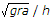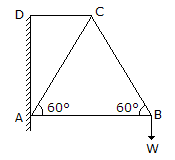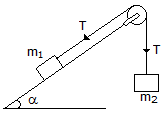Courses

# Test: Engineering Mechanics - 3

## 25 Questions MCQ Test Engineering Mechanics | Test: Engineering Mechanics - 3

Description
This mock test of Test: Engineering Mechanics - 3 for Mechanical Engineering helps you for every Mechanical Engineering entrance exam. This contains 25 Multiple Choice Questions for Mechanical Engineering Test: Engineering Mechanics - 3 (mcq) to study with solutions a complete question bank. The solved questions answers in this Test: Engineering Mechanics - 3 quiz give you a good mix of easy questions and tough questions. Mechanical Engineering students definitely take this Test: Engineering Mechanics - 3 exercise for a better result in the exam. You can find other Test: Engineering Mechanics - 3 extra questions, long questions & short questions for Mechanical Engineering on EduRev as well by searching above.
QUESTION: 1

Solution:
QUESTION: 2

Solution:
QUESTION: 3

### The overturning of a vehicle on a level circular path can be avoided if the velocity of vehicle is __________.

Solution:
QUESTION: 4

Which of the following is a scalar quantity?

Solution:
QUESTION: 5

The rate of change of momentum is directly proportional to the impressed force, and takes place in the same direction in which the force acts. This statement is known as

Solution:
QUESTION: 6

In ideal machines, mechanical advantage is __________ velocity ratio.

Solution:
QUESTION: 7

The minimum force required to slide a body of weight W on a rough horizontal plane is

Solution:
QUESTION: 8

The moment of inertia of a square of side a about its diagonal is

Solution:
QUESTION: 9

The angular velocity (in rad / s) of a body rotating at N revolutions per minute is

Solution:
QUESTION: 10

The maximum efficiency of a lifting machine is

Solution:
QUESTION: 11

The centre of gravity of a semi-circle lies at a distance of __________ from its base measured along the vertical radius.

Solution:
QUESTION: 12

The process of finding out the resultant force is called __________ of forces.

Solution:
QUESTION: 13

A weight of 1000 N can be lifted by an effort of 80 N. If the velocity ratio is 20, the machine is

Solution:
QUESTION: 14

In a framed structure, as shown in the below figure, the force in the member BC isSolution:
QUESTION: 15

The potential energy of a vertically raised body is __________ the kinetic energy of a vertically falling body.

Solution:
QUESTION: 16

If a number of forces acting at a point be represented in magnitude and direction by the three sides of a triangle, taken in order, then the forces are not in equilibrium.

Solution:
QUESTION: 17

The periodic time of a particle with simple harmonic motion is __________ proportional to the angular velocity.

Solution:
QUESTION: 18

The motion of a particle round a fixed axis is

Solution:
QUESTION: 19

A block of mass m1, placed on an inclined smooth plane is connected by a light string passing over a smooth pulley to mass m2, which moves vertically downwards as shown in the below figure. The tension in the string isSolution:
QUESTION: 20

If a given force (or a given system of forces) acting on a body __________ the position of the body, but keeps it in equilibrium, then its effect is to produce internal stress in the body.

Solution:
QUESTION: 21

The principle of transmissibility of forces states that, when a force acts upon a body, its effect is

Solution:
QUESTION: 22

A differential pulley block has larger and smaller diameters of 100 mm and 80 mm respectively. Its velocity ratio is

Solution:
QUESTION: 23

If three forces acting at a point are represented in magnitude and direction by the three sides of a triangle, taken in order, then the forces are in equilibrium.

Solution:
QUESTION: 24

Newton's second law motion __________ a relation between force and mass of a moving body.

Solution:
QUESTION: 25

The periodic time of one oscillation for a simple pendulum is(where l = Length of the pendulum.)

Solution: